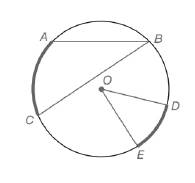Chapter 6.1, Problem 8EElementary Geometry For College St...

7th Edition
Alexander + 2 others
ISBN: 9781337614085

Solutions

Chapter
SectionElementary Geometry For College St...

7th Edition
Alexander + 2 others
ISBN: 9781337614085
Textbook Problem

For Exercises 1 to 8, use the figure provided.Exercises 1-8If m E C A B D ⌢ = 312 ∘ , find m ∠ D O E .

To determine

To calculate:

The mDOE, if mECABD=312 by using the provided figure.

Explanation

Given:

The provided figure is

Postulate used:

Central angle postulate:

In a circle, the degree measure of a central angle is equal to the degree measure of its intercepted arc.

Calculation:

The intercepted arc of DOE is DE.

From the figure, we see that the arcs DE,ECABD form the whole circle.

Therefore mDE+mECABD=360

Substitute mECABD=312 in mDE+mECABD=360

Still sussing out bartleby?

Check out a sample textbook solution.

See a sample solution

The Solution to Your Study Problems

Bartleby provides explanations to thousands of textbook problems written by our experts, many with advanced degrees!

Get Started

Fill in each blank: 4qt1pt=pt

Elementary Technical Mathematics

Expand each expression in Exercises 122. (4+2x)(42x)

Finite Mathematics and Applied Calculus (MindTap Course List)

A definite integral for the length of y = x3, 1 x 2 is a) 121+x3dx b) 121+x6dx c) 121+9x4dx d) 121+3x2dx

Study Guide for Stewart's Single Variable Calculus: Early Transcendentals, 8th

The graph of x = cos t, y = sin2 t is:

Study Guide for Stewart's Multivariable Calculus, 8th

Define induction and deduction and explain the role of each in the scientific method.

Research Methods for the Behavioral Sciences (MindTap Course List)